An Intro to DifferentialEquations.jl

Basic Introduction Via Ordinary Differential Equations

This notebook will get you started with DifferentialEquations.jl by introducing you to the functionality for solving ordinary differential equations (ODEs). The corresponding documentation page is the ODE tutorial. While some of the syntax may be different for other types of equations, the same general principles hold in each case. Our goal is to give a gentle and thorough introduction that highlights these principles in a way that will help you generalize what you have learned.

Background

If you are new to the study of differential equations, it can be helpful to do a quick background read on the definition of ordinary differential equations. We define an ordinary differential equation as an equation which describes the way that a variable $u$ changes, that is

$u' = f(u,p,t)$

where $p$ are the parameters of the model, $t$ is the time variable, and $f$ is the nonlinear model of how $u$ changes. The initial value problem also includes the information about the starting value:

$u(t_0) = u_0$

Together, if you know the starting value and you know how the value will change with time, then you know what the value will be at any time point in the future. This is the intuitive definition of a differential equation.

First Model: Exponential Growth

Our first model will be the canonical exponential growth model. This model says that the rate of change is proportional to the current value, and is this:

$u' = au$

where we have a starting value $u(0)=u_0$. Let's say we put 1 dollar into Bitcoin which is increasing at a rate of $98\%$ per year. Then calling now $t=0$ and measuring time in years, our model is:

$u' = 0.98u$

and $u(0) = 1.0$. We encode this into Julia by noticing that, in this setup, we match the general form when

f(u,p,t) = 0.98u
f (generic function with 1 method)

with $u_0 = 1.0$. If we want to solve this model on a time span from t=0.0 to t=1.0, then we define an ODEProblem by specifying this function f, this initial condition u0, and this time span as follows:

using DifferentialEquations
f(u,p,t) = 0.98u
u0 = 1.0
tspan = (0.0,1.0)
prob = ODEProblem(f,u0,tspan)
ODEProblem with uType Float64 and tType Float64. In-place: false
timespan: (0.0, 1.0)
u0: 1.0

To solve our ODEProblem we use the command solve.

sol = solve(prob)
retcode: Success
Interpolation: Automatic order switching interpolation
t: 5-element Array{Float64,1}:
0.0
0.10042494449239292
0.3521855598485865
0.6934428591625682
1.0
u: 5-element Array{Float64,1}:
1.0
1.1034222047865465
1.4121902209793713
1.9730369896422575
2.664456142481387

and that's it: we have succesfully solved our first ODE!

Analyzing the Solution

Of course, the solution type is not interesting in and of itself. We want to understand the solution! The documentation page which explains in detail the functions for analyzing the solution is the Solution Handling page. Here we will describe some of the basics. You can plot the solution using the plot recipe provided by Plots.jl:

using Plots; gr()
plot(sol)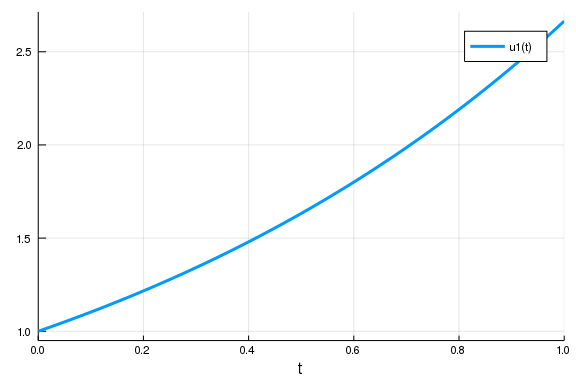From the picture we see that the solution is an exponential curve, which matches our intuition. As a plot recipe, we can annotate the result using any of the Plots.jl attributes. For example:

plot(sol,linewidth=5,title="Solution to the linear ODE with a thick line",
xaxis="Time (t)",yaxis="u(t) (in μm)",label="My Thick Line!") # legend=false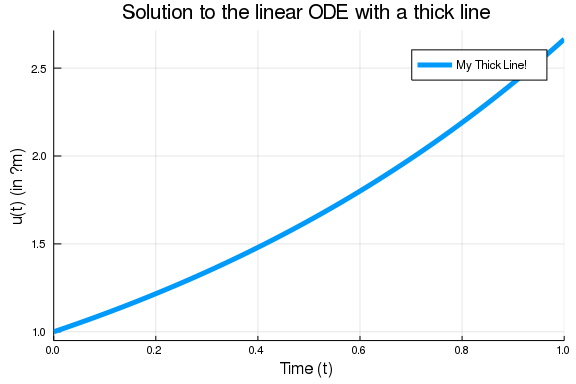Using the mutating plot! command we can add other pieces to our plot. For this ODE we know that the true solution is $u(t) = u_0 exp(at)$, so let's add some of the true solution to our plot:

plot!(sol.t, t->1.0*exp(0.98t),lw=3,ls=:dash,label="True Solution!")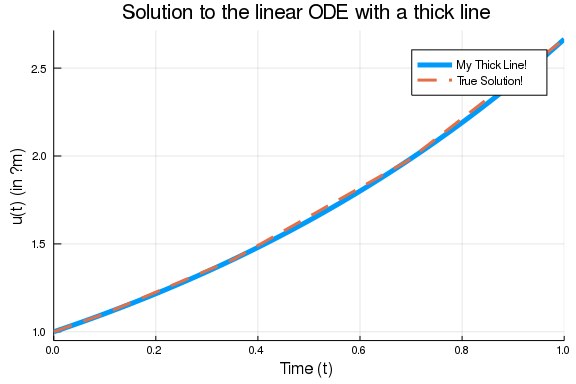In the previous command I demonstrated sol.t, which grabs the array of time points that the solution was saved at:

sol.t
5-element Array{Float64,1}:
0.0
0.10042494449239292
0.3521855598485865
0.6934428591625682
1.0

We can get the array of solution values using sol.u:

sol.u
5-element Array{Float64,1}:
1.0
1.1034222047865465
1.4121902209793713
1.9730369896422575
2.664456142481387

sol.u[i] is the value of the solution at time sol.t[i]. We can compute arrays of functions of the solution values using standard comprehensions, like:

[t+u for (u,t) in tuples(sol)]
5-element Array{Float64,1}:
1.0
1.2038471492789395
1.7643757808279579
2.666479848804826
3.664456142481387

However, one interesting feature is that, by default, the solution is a continuous function. If we check the print out again:

sol
retcode: Success
Interpolation: Automatic order switching interpolation
t: 5-element Array{Float64,1}:
0.0
0.10042494449239292
0.3521855598485865
0.6934428591625682
1.0
u: 5-element Array{Float64,1}:
1.0
1.1034222047865465
1.4121902209793713
1.9730369896422575
2.664456142481387

you see that it says that the solution has a order changing interpolation. The default algorithm automatically switches between methods in order to handle all types of problems. For non-stiff equations (like the one we are solving), it is a continuous function of 4th order accuracy. We can call the solution as a function of time sol(t). For example, to get the value at t=0.45, we can use the command:

sol(0.45)
1.5542610480525971

Controlling the Solver

DifferentialEquations.jl has a common set of solver controls among its algorithms which can be found at the Common Solver Options page. We will detail some of the most widely used options.

The most useful options are the tolerances abstol and reltol. These tell the internal adaptive time stepping engine how precise of a solution you want. Generally, reltol is the relative accuracy while abstol is the accuracy when u is near zero. These tolerances are local tolerances and thus are not global guarantees. However, a good rule of thumb is that the total solution accuracy is 1-2 digits less than the relative tolerances. Thus for the defaults abstol=1e-6 and reltol=1e-3, you can expect a global accuracy of about 1-2 digits. If we want to get around 6 digits of accuracy, we can use the commands:

sol = solve(prob,abstol=1e-8,reltol=1e-8)
retcode: Success
Interpolation: Automatic order switching interpolation
t: 9-element Array{Float64,1}:
0.0
0.04127492324135852
0.14679466086219672
0.2863090396112191
0.438184089090746
0.6118802875301362
0.7985514876572974
0.9993352795953876
1.0
u: 9-element Array{Float64,1}:
1.0
1.0412786454705882
1.1547210130399164
1.32390123501071
1.5363667984773475
1.8214678404507973
2.187108732054802
2.66272111108696
2.6644562419335163

Now we can see no visible difference against the true solution:

plot(sol)
plot!(sol.t, t->1.0*exp(0.98t),lw=3,ls=:dash,label="True Solution!")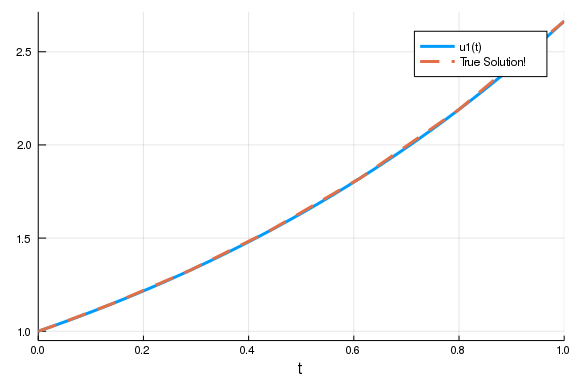Notice that by decreasing the tolerance, the number of steps the solver had to take was 9 instead of the previous 5. There is a trade off between accuracy and speed, and it is up to you to determine what is the right balance for your problem.

Another common option is to use saveat to make the solver save at specific time points. For example, if we want the solution at an even grid of t=0.1k for integers k, we would use the command:

sol = solve(prob,saveat=0.1)
retcode: Success
Interpolation: 1st order linear
t: 11-element Array{Float64,1}:
0.0
0.1
0.2
0.3
0.4
0.5
0.6
0.7
0.8
0.9
1.0
u: 11-element Array{Float64,1}:
1.0
1.1029627851292922
1.2165269512231858
1.3417838212289122
1.479937951060823
1.63231620704857
1.8003833265032916
1.9857565541611835
2.1902158127993507
2.41572574207719
2.664456142481387

Notice that when saveat is used the continuous output variables are no longer saved and thus sol(t), the interpolation, is only first order. We can save at an uneven grid of points by passing a collection of values to saveat. For example:

sol = solve(prob,saveat=[0.2,0.7,0.9])
retcode: Success
Interpolation: 1st order linear
t: 3-element Array{Float64,1}:
0.2
0.7
0.9
u: 3-element Array{Float64,1}:
1.2165269512231858
1.9857565541611835
2.41572574207719

If we need to reduce the amount of saving, we can also turn off the continuous output directly via dense=false:

sol = solve(prob,dense=false)
retcode: Success
Interpolation: 1st order linear
t: 5-element Array{Float64,1}:
0.0
0.10042494449239292
0.3521855598485865
0.6934428591625682
1.0
u: 5-element Array{Float64,1}:
1.0
1.1034222047865465
1.4121902209793713
1.9730369896422575
2.664456142481387

and to turn off all intermediate saving we can use save_everystep=false:

sol = solve(prob,save_everystep=false)
retcode: Success
Interpolation: 1st order linear
t: 2-element Array{Float64,1}:
0.0
1.0
u: 2-element Array{Float64,1}:
1.0
2.664456142481387

If we want to solve and only save the final value, we can even set save_start=false.

sol = solve(prob,save_everystep=false,save_start = false)
retcode: Success
Interpolation: 1st order linear
t: 1-element Array{Float64,1}:
1.0
u: 1-element Array{Float64,1}:
2.664456142481387

Note that similarly on the other side there is save_end=false.

More advanced saving behaviors, such as saving functionals of the solution, are handled via the SavingCallback in the Callback Library which will be addressed later in the tutorial.

Choosing Solver Algorithms

There is no best algorithm for numerically solving a differential equation. When you call solve(prob), DifferentialEquations.jl makes a guess at a good algorithm for your problem, given the properties that you ask for (the tolerances, the saving information, etc.). However, in many cases you may want more direct control. A later notebook will help introduce the various algorithms in DifferentialEquations.jl, but for now let's introduce the syntax.

The most crucial determining factor in choosing a numerical method is the stiffness of the model. Stiffness is roughly characterized by a Jacobian f with large eigenvalues. That's quite mathematical, and we can think of it more intuitively: if you have big numbers in f (like parameters of order 1e5), then it's probably stiff. Or, as the creator of the MATLAB ODE Suite, Lawrence Shampine, likes to define it, if the standard algorithms are slow, then it's stiff. We will go into more depth about diagnosing stiffness in a later tutorial, but for now note that if you believe your model may be stiff, you can hint this to the algorithm chooser via alg_hints = [:stiff].

sol = solve(prob,alg_hints=[:stiff])
retcode: Success
Interpolation: specialized 3rd order "free" stiffness-aware interpolation
t: 8-element Array{Float64,1}:
0.0
0.05653299582822294
0.17270897997721946
0.3164619936069947
0.5057530766813646
0.7292290122455201
0.9913056881982787
1.0
u: 8-element Array{Float64,1}:
1.0
1.0569657840332976
1.184421874952142
1.3636060527266576
1.6415448917417383
2.0434588086024563
2.641846814956192
2.664452642975646

Stiff algorithms have to solve implicit equations and linear systems at each step so they should only be used when required.

If we want to choose an algorithm directly, you can pass the algorithm type after the problem as solve(prob,alg). For example, let's solve this problem using the Tsit5() algorithm, and just for show let's change the relative tolerance to 1e-6 at the same time:

sol = solve(prob,Tsit5(),reltol=1e-6)
retcode: Success
Interpolation: specialized 4th order "free" interpolation
t: 10-element Array{Float64,1}:
0.0
0.028970819746309166
0.10049166978837214
0.19458902376186224
0.3071721467343173
0.43945340580499864
0.5883428480879211
0.7524861839187198
0.9293007851261506
1.0
u: 10-element Array{Float64,1}:
1.0
1.0287982807225062
1.103494360777622
1.2100930328474355
1.3512481270061714
1.5382791211530558
1.7799334774107156
2.0905693823853637
2.486098887385528
2.6644562434913315

Systems of ODEs: The Lorenz Equation

Now let's move to a system of ODEs. The Lorenz equation is the famous "butterfly attractor" that spawned chaos theory. It is defined by the system of ODEs:

\begin{align} \frac{dx}{dt} &= \sigma (y - x)\\ \frac{dy}{dt} &= x (\rho - z) -y\\ \frac{dz}{dt} &= xy - \beta z \end{align}

To define a system of differential equations in DifferentialEquations.jl, we define our f as a vector function with a vector initial condition. Thus, for the vector u = [x,y,z]', we have the derivative function:

function lorenz!(du,u,p,t)
σ,ρ,β = p
du = σ*(u-u)
du = u*(ρ-u) - u
du = u*u - β*u
end
lorenz! (generic function with 1 method)

Notice here we used the in-place format which writes the output to the preallocated vector du. For systems of equations the in-place format is faster. We use the initial condition $u_0 = [1.0,0.0,0.0]$ as follows:

u0 = [1.0,0.0,0.0]
3-element Array{Float64,1}:
1.0
0.0
0.0

Lastly, for this model we made use of the parameters p. We need to set this value in the ODEProblem as well. For our model we want to solve using the parameters $\sigma = 10$, $\rho = 28$, and $\beta = 8/3$, and thus we build the parameter collection:

p = (10,28,8/3) # we could also make this an array, or any other type!
(10, 28, 2.6666666666666665)

Now we generate the ODEProblem type. In this case, since we have parameters, we add the parameter values to the end of the constructor call. Let's solve this on a time span of t=0 to t=100:

tspan = (0.0,100.0)
prob = ODEProblem(lorenz!,u0,tspan,p)
ODEProblem with uType Array{Float64,1} and tType Float64. In-place: true
timespan: (0.0, 100.0)
u0: [1.0, 0.0, 0.0]

Now, just as before, we solve the problem:

sol = solve(prob)
retcode: Success
Interpolation: Automatic order switching interpolation
t: 1250-element Array{Float64,1}:
0.0
3.5678604836301404e-5
0.0003924646531993154
0.0032623883208835647
0.00905805935549092
0.016956466266909925
0.027689961278342563
0.041856290192165115
0.06024018681535046
0.0836851555247397
⋮
99.50064429327207
99.56497345673458
99.62788705014984
99.6991013016854
99.75654880247485
99.81017638953824
99.87131062092273
99.93558797583201
100.0
u: 1250-element Array{Array{Float64,1},1}:
[1.0, 0.0, 0.0]
[0.999643, 0.000998805, 1.78143e-8]
[0.996105, 0.0109654, 2.14696e-6]
[0.969359, 0.0897701, 0.0001438]
[0.924204, 0.242289, 0.00104616]
[0.880046, 0.438736, 0.00342426]
[0.848331, 0.691563, 0.00848763]
[0.849504, 1.01454, 0.018212]
[0.913906, 1.44255, 0.0366935]
[1.08886, 2.05232, 0.0740252]
⋮
[8.87662, 1.1596, 35.1377]
[4.55579, -0.800246, 29.5784]
[2.06483, -0.641055, 24.865]
[0.835596, -0.129419, 20.5289]
[0.493369, 0.189755, 17.614]
[0.425759, 0.448441, 15.274]
[0.520676, 0.802846, 12.9926]
[0.797852, 1.39909, 10.9881]
[1.34105, 2.47931, 9.37471]

The same solution handling features apply to this case. Thus sol.t stores the time points and sol.u is an array storing the solution at the corresponding time points.

However, there are a few extra features which are good to know when dealing with systems of equations. First of all, sol also acts like an array. sol[i] returns the solution at the ith time point.

sol.t,sol
(0.0836851555247397, [1.08886, 2.05232, 0.0740252])

Additionally, the solution acts like a matrix where sol[j,i] is the value of the jth variable at time i:

sol[2,10]
2.0523193075036916

We can get a real matrix by performing a conversion:

A = Array(sol)
3×1250 Array{Float64,2}:
1.0  0.999643     0.996105    0.969359   …   0.520676   0.797852  1.34105
0.0  0.000998805  0.0109654   0.0897701      0.802846   1.39909   2.47931
0.0  1.78143e-8   2.14696e-6  0.0001438     12.9926    10.9881    9.37471

This is the same as sol, i.e. sol[i,j] = A[i,j], but now it's a true matrix. Plotting will by default show the time series for each variable:

plot(sol)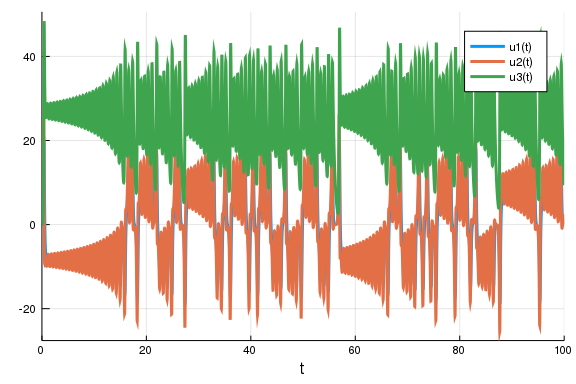If we instead want to plot values against each other, we can use the vars command. Let's plot variable 1 against variable 2 against variable 3:

plot(sol,vars=(1,2,3))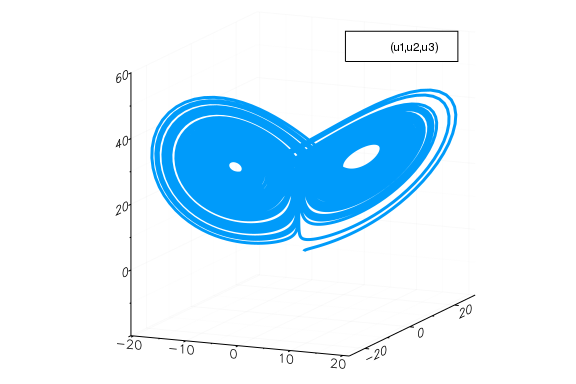This is the classic Lorenz attractor plot, where the x axis is u, the y axis is u, and the z axis is u. Note that the plot recipe by default uses the interpolation, but we can turn this off:

plot(sol,vars=(1,2,3),denseplot=false)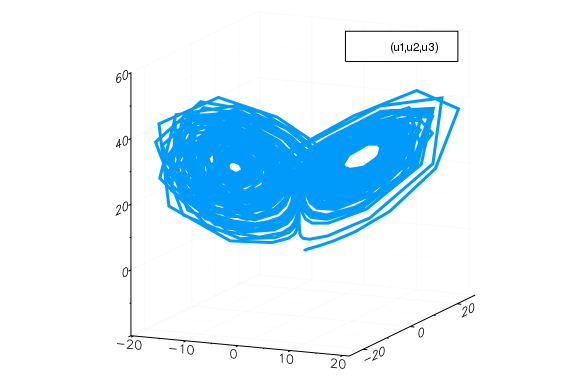Yikes! This shows how calculating the continuous solution has saved a lot of computational effort by computing only a sparse solution and filling in the values! Note that in vars, 0=time, and thus we can plot the time series of a single component like:

plot(sol,vars=(0,2))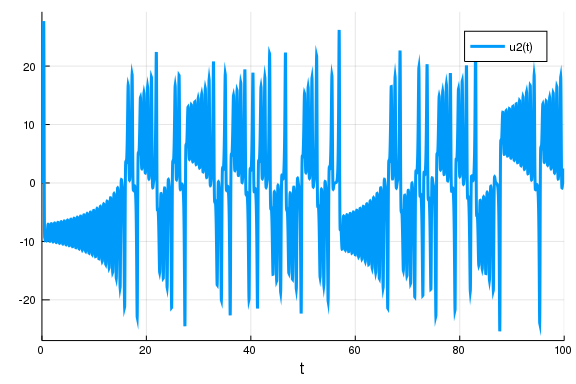A DSL for Parameterized Functions

In many cases you may be defining a lot of functions with parameters. There exists the domain-specific language (DSL) defined by the @ode_def macro for helping with this common problem. For example, we can define the Lotka-Volterra equation:

\begin{align} \frac{dx}{dt} &= ax - bxy\\ \frac{dy}{dt} &= -cy + dxy \end{align}

as follows:

function lotka_volterra!(du,u,p,t)
du = p*u - p*u*u
du = -p*u + p*u*u
end
lotka_volterra! (generic function with 1 method)

However, that can be hard to follow since there's a lot of "programming" getting in the way. Instead, you can use the @ode_def macro from ParameterizedFunctions.jl:

using ParameterizedFunctions
lv! = @ode_def LotkaVolterra begin
dx = a*x - b*x*y
dy = -c*y + d*x*y
end a b c d
(::Main.WeaveSandBox2.LotkaVolterra{getfield(Main.WeaveSandBox2, Symbol("##
7#11")),getfield(Main.WeaveSandBox2, Symbol("##8#12")),getfield(Main.WeaveS
andBox2, Symbol("##9#13")),Nothing,Nothing,getfield(Main.WeaveSandBox2, Sym
bol("##10#14")),Expr,Expr}) (generic function with 2 methods)

We can then use the result just like an ODE function from before:

u0 = [1.0,1.0]
p = (1.5,1.0,3.0,1.0)
tspan = (0.0,10.0)
prob = ODEProblem(lv!,u0,tspan,p)
sol = solve(prob)
plot(sol)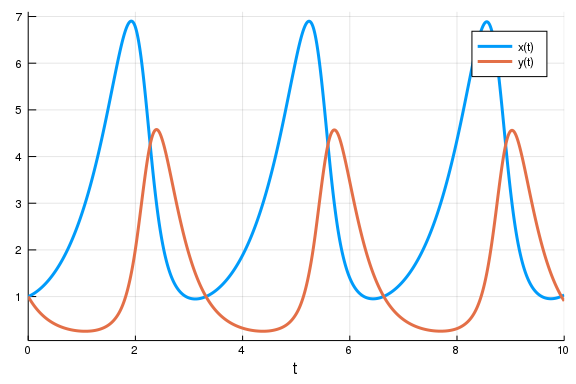Not only is the DSL convenient syntax, but it does some magic behind the scenes. For example, further parts of the tutorial will describe how solvers for stiff differential equations have to make use of the Jacobian in calculations. Here, the DSL uses symbolic differentiation to automatically derive that function:

lv!.Jex
quote
internal_var___J[1, 1] = internal_var___p - internal_var___p * in
ternal_var___u
internal_var___J[1, 2] = -(internal_var___p) * internal_var___u
internal_var___J[2, 1] = internal_var___p * internal_var___u
internal_var___J[2, 2] = -(internal_var___p) + internal_var___p *
internal_var___u
nothing
end

The DSL can derive many other functions; this ability is used to speed up the solvers. An extension to DifferentialEquations.jl, Latexify.jl, allows you to extract these pieces as LaTeX expressions.

Internal Types

The last basic user-interface feature to explore is the choice of types. DifferentialEquations.jl respects your input types to determine the internal types that are used. Thus since in the previous cases, when we used Float64 values for the initial condition, this meant that the internal values would be solved using Float64. We made sure that time was specified via Float64 values, meaning that time steps would utilize 64-bit floats as well. But, by simply changing these types we can change what is used internally.

As a quick example, let's say we want to solve an ODE defined by a matrix. To do this, we can simply use a matrix as input.

A  = [1. 0  0 -5
4 -2  4 -3
-4  0  0  1
5 -2  2  3]
u0 = rand(4,2)
tspan = (0.0,1.0)
f(u,p,t) = A*u
prob = ODEProblem(f,u0,tspan)
sol = solve(prob)
retcode: Success
Interpolation: Automatic order switching interpolation
t: 10-element Array{Float64,1}:
0.0
0.04330119198184582
0.10983948286225614
0.18801803393561917
0.2866240584151791
0.40906989372159136
0.5511592223989411
0.704033661613616
0.8632290632699496
1.0
u: 10-element Array{Array{Float64,2},1}:
[0.425892 0.260488; 0.428294 0.452756; 0.440276 0.234219; 0.972781 0.96522
3]
[0.205955 0.0411449; 0.382764 0.350298; 0.431521 0.252682; 1.1843 1.11992]

[-0.237451 -0.376748; 0.199172 0.112611; 0.521557 0.376699; 1.47012 1.3198
]
[-0.908936 -0.979168; -0.153697 -0.253996; 0.82237 0.695871; 1.72456 1.479
84]
[-1.94502 -1.86916; -0.70898 -0.759724; 1.5587 1.40392; 1.87843 1.53404]

[-3.39785 -3.06027; -1.3259 -1.24474; 3.08878 2.78851; 1.73244 1.31272]

[-5.00169 -4.28837; -1.45563 -1.20686; 5.69091 5.03387; 0.978203 0.569233]

[-6.04268 -4.92898; -0.180054 0.118958; 9.1593 7.88829; -0.644615 -0.88556
8]
[-5.50386 -4.13384; 3.45481 3.46671; 12.6522 10.5655; -3.233 -3.09436]

[-2.96499 -1.66434; 8.7865 8.1325; 14.443 11.6611; -6.02482 -5.39004]

There is no real difference from what we did before, but now in this case u0 is a 4x2 matrix. Because of that, the solution at each time point is matrix:

sol
4×2 Array{Float64,2}:
-0.237451  -0.376748
0.199172   0.112611
0.521557   0.376699
1.47012    1.3198

In DifferentialEquations.jl, you can use any type that defines +, -, *, /, and has an appropriate norm. For example, if we want arbitrary precision floating point numbers, we can change the input to be a matrix of BigFloat:

big_u0 = big.(u0)
4×2 Array{BigFloat,2}:
0.425892  0.260488
0.428294  0.452756
0.440276  0.234219
0.972781  0.965223

and we can solve the ODEProblem with arbitrary precision numbers by using that initial condition:

prob = ODEProblem(f,big_u0,tspan)
sol = solve(prob)
retcode: Success
Interpolation: Automatic order switching interpolation
t: 6-element Array{Float64,1}:
0.0
0.12950311238875903
0.3776286867278208
0.6826307059183888
0.9970081360265544
1.0
u: 6-element Array{Array{BigFloat,2},1}:
[0.425892 0.260488; 0.428294 0.452756; 0.440276 0.234219; 0.972781 0.96522
3]
[-0.391808 -0.517723; 0.122299 0.0273673; 0.575874 0.438266; 1.54336 1.368
42]
[-3.01811 -2.75499; -1.19358 -1.1508; 2.62955 2.38001; 1.81053 1.40378]

[-5.96797 -4.90523; -0.474788 -0.168012; 8.65576 7.48346; -0.36485 -0.6404
1]
[-3.04563 -1.73948; 8.64814 8.01353; 14.425 11.6568; -5.9603 -5.33791]

[-2.96497 -1.66433; 8.78654 8.13253; 14.4431 11.6611; -6.02484 -5.39005]
sol[1,3]
-3.018112846173883596414677502392249031603692933761110249554752186715212119
276106

To really make use of this, we would want to change abstol and reltol to be small! Notice that the type for "time" is different than the type for the dependent variables, and this can be used to optimize the algorithm via keeping multiple precisions. We can convert time to be arbitrary precision as well by defining our time span with BigFloat variables:

prob = ODEProblem(f,big_u0,big.(tspan))
sol = solve(prob)
retcode: Success
Interpolation: Automatic order switching interpolation
t: 6-element Array{BigFloat,1}:
0.0

0.129503112388759025420352070500060404254736837969783646324403117273289130
0107554
0.377628686727820828392763533162396453198998194550909011512149758140026290
6102944
0.682630705918388891886570361685113156259420847153670634093012746413852003
6864237
0.997008136026554409331084788629910535308360292831171029276156141723398349
3437613
1.0

u: 6-element Array{Array{BigFloat,2},1}:
[0.425892 0.260488; 0.428294 0.452756; 0.440276 0.234219; 0.972781 0.96522
3]
[-0.391808 -0.517723; 0.122299 0.0273673; 0.575874 0.438266; 1.54336 1.368
42]
[-3.01811 -2.75499; -1.19358 -1.1508; 2.62955 2.38001; 1.81053 1.40378]

[-5.96797 -4.90523; -0.474788 -0.168012; 8.65576 7.48346; -0.36485 -0.6404
1]
[-3.04563 -1.73948; 8.64814 8.01353; 14.425 11.6568; -5.9603 -5.33791]

[-2.96497 -1.66433; 8.78654 8.13253; 14.4431 11.6611; -6.02484 -5.39005]

Let's end by showing a more complicated use of types. For small arrays, it's usually faster to do operations on static arrays via the package StaticArrays.jl. The syntax is similar to that of normal arrays, but for these special arrays we utilize the @SMatrix macro to indicate we want to create a static array.

using StaticArrays
A  = @SMatrix [ 1.0  0.0 0.0 -5.0
4.0 -2.0 4.0 -3.0
-4.0  0.0 0.0  1.0
5.0 -2.0 2.0  3.0]
u0 = @SMatrix rand(4,2)
tspan = (0.0,1.0)
f(u,p,t) = A*u
prob = ODEProblem(f,u0,tspan)
sol = solve(prob)
retcode: Success
Interpolation: Automatic order switching interpolation
t: 11-element Array{Float64,1}:
0.0
0.0336160180207602
0.08412997362621587
0.14806631456943903
0.22433133614380857
0.31990966213948546
0.4400739108897834
0.5832156804749542
0.7416649809662037
0.9061477391769605
1.0
u: 11-element Array{StaticArrays.SArray{Tuple{4,2},Float64,2,8},1}:
[0.43626 0.915419; 0.891509 0.380879; 0.0155531 0.332733; 0.516733 0.98606
2]
[0.357329 0.757107; 0.831212 0.394842; -0.0194905 0.257073; 0.580784 1.232
47]
[0.214081 0.43128; 0.709436 0.311625; -0.0461453 0.206495; 0.666519 1.5845
5]
[-0.006741 -0.130363; 0.510945 0.0442428; -0.0280178 0.278947; 0.753493 1.
98499]
[-0.320198 -1.00634; 0.230087 -0.465588; 0.0808634 0.613692; 0.819292 2.36
965]
[-0.769708 -2.37667; -0.143448 -1.27988; 0.36721 1.49398; 0.831372 2.65613
]
[-1.37031 -4.38711; -0.543736 -2.2827; 0.975863 3.43225; 0.713097 2.6038]

[-2.00833 -6.81153; -0.718739 -2.79939; 2.03013 6.97404; 0.344448 1.76747]

[-2.36988 -8.71156; -0.235017 -1.44401; 3.44643 12.0958; -0.380711 -0.3405
71]
[-2.00395 -8.5107; 1.30505 3.32618; 4.78979 17.5928; -1.46597 -3.91971]

[-1.3054 -6.80442; 2.70972 7.9245; 5.25233 20.0236; -2.19586 -6.51796]
sol
4×2 StaticArrays.SArray{Tuple{4,2},Float64,2,8}:
0.214081   0.43128
0.709436   0.311625
-0.0461453  0.206495
0.666519   1.58455

Conclusion

These are the basic controls in DifferentialEquations.jl. All equations are defined via a problem type, and the solve command is used with an algorithm choice (or the default) to get a solution. Every solution acts the same, like an array sol[i] with sol.t[i], and also like a continuous function sol(t) with a nice plot command plot(sol). The Common Solver Options can be used to control the solver for any equation type. Lastly, the types used in the numerical solving are determined by the input types, and this can be used to solve with arbitrary precision and add additional optimizations (this can be used to solve via GPUs for example!). While this was shown on ODEs, these techniques generalize to other types of equations as well.

Appendix

This tutorial is part of the DiffEqTutorials.jl repository, found at: https://github.com/JuliaDiffEq/DiffEqTutorials.jl

To locally run this tutorial, do the following commands:

using DiffEqTutorials
DiffEqTutorials.weave_file("introduction","01-ode_introduction.jmd")

Computer Information:

Julia Version 1.1.1
Commit 55e36cc308 (2019-05-16 04:10 UTC)
Platform Info:
OS: Linux (x86_64-pc-linux-gnu)
CPU: Intel(R) Core(TM) i7-3770 CPU @ 3.40GHz
WORD_SIZE: 64
LIBM: libopenlibm
LLVM: libLLVM-6.0.1 (ORCJIT, ivybridge)

Package Information:

Status ~/.julia/environments/v1.1/Project.toml
[7e558dbc-694d-5a72-987c-6f4ebed21442] ArbNumerics 0.5.4
[6e4b80f9-dd63-53aa-95a3-0cdb28fa8baf] BenchmarkTools 0.4.2
[be33ccc6-a3ff-5ff2-a52e-74243cff1e17] CUDAnative 2.2.0
[3a865a2d-5b23-5a0f-bc46-62713ec82fae] CuArrays 1.0.2
[55939f99-70c6-5e9b-8bb0-5071ed7d61fd] DecFP 0.4.8
[abce61dc-4473-55a0-ba07-351d65e31d42] Decimals 0.4.0
[ebbdde9d-f333-5424-9be2-dbf1e9acfb5e] DiffEqBayes 1.1.0
[eb300fae-53e8-50a0-950c-e21f52c2b7e0] DiffEqBiological 3.8.2
[459566f4-90b8-5000-8ac3-15dfb0a30def] DiffEqCallbacks 2.5.2
[f3b72e0c-5b89-59e1-b016-84e28bfd966d] DiffEqDevTools 2.9.0
[1130ab10-4a5a-5621-a13d-e4788d82bd4c] DiffEqParamEstim 1.6.0
[055956cb-9e8b-5191-98cc-73ae4a59e68a] DiffEqPhysics 3.1.0
[6d1b261a-3be8-11e9-3f2f-0b112a9a8436] DiffEqTutorials 0.1.0
[0c46a032-eb83-5123-abaf-570d42b7fbaa] DifferentialEquations 6.4.0
[31c24e10-a181-5473-b8eb-7969acd0382f] Distributions 0.20.0
[497a8b3b-efae-58df-a0af-a86822472b78] DoubleFloats 0.9.1
[f6369f11-7733-5829-9624-2563aa707210] ForwardDiff 0.10.3
[4138dd39-2aa7-5051-a626-17a0bb65d9c8] JLD 0.9.1
[23fbe1c1-3f47-55db-b15f-69d7ec21a316] Latexify 0.8.2
[eff96d63-e80a-5855-80a2-b1b0885c5ab7] Measurements 2.0.0
[961ee093-0014-501f-94e3-6117800e7a78] ModelingToolkit 0.2.0
[76087f3c-5699-56af-9a33-bf431cd00edd] NLopt 0.5.1
[2774e3e8-f4cf-5e23-947b-6d7e65073b56] NLsolve 4.0.0
[429524aa-4258-5aef-a3af-852621145aeb] Optim 0.18.1
[1dea7af3-3e70-54e6-95c3-0bf5283fa5ed] OrdinaryDiffEq 5.8.1
[65888b18-ceab-5e60-b2b9-181511a3b968] ParameterizedFunctions 4.1.1
[91a5bcdd-55d7-5caf-9e0b-520d859cae80] Plots 0.25.1
[d330b81b-6aea-500a-939a-2ce795aea3ee] PyPlot 2.8.1
[731186ca-8d62-57ce-b412-fbd966d074cd] RecursiveArrayTools 0.20.0
[90137ffa-7385-5640-81b9-e52037218182] StaticArrays 0.11.0
[f3b207a7-027a-5e70-b257-86293d7955fd] StatsPlots 0.11.0